# Grade 4 Math STAAR Review Activities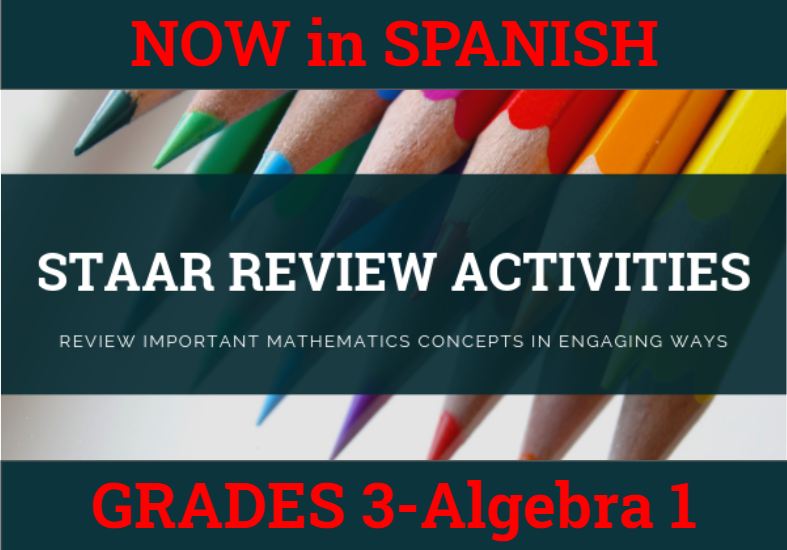How might we purposefully and effectively review important mathematics concepts for the Grade 4 Math STAAR assessment? The ESC-18 Math Team has created a variety of activities where students practice and apply important grade-level TEKS aligned topics to cement their learning.

### Please See Our Pricing and Instructions to Purchase our STAAR Activities

 4.2B Representing Place Value Quick Flips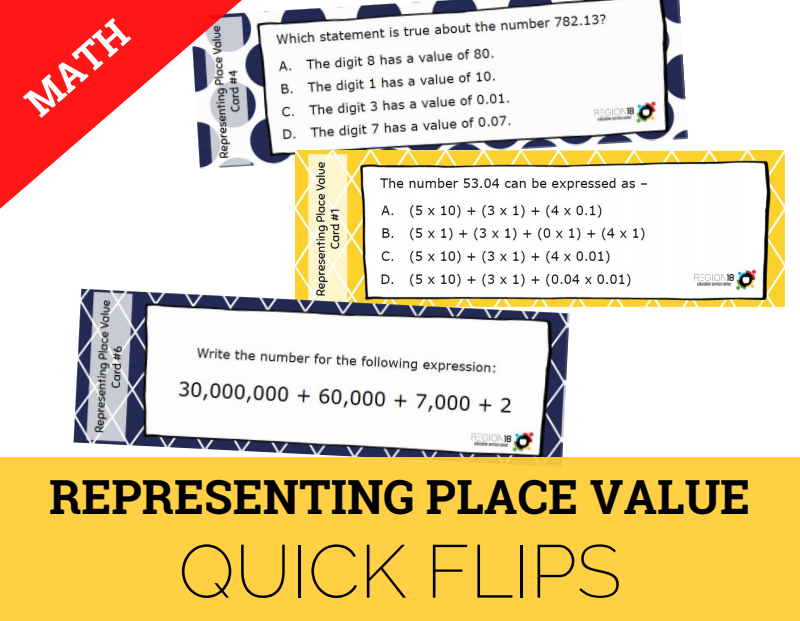Represent the value of the digit in whole numbers through 1,000,000,000 and decimals to the hundredths using expanded notation and numerals. 4.2G Decimal Representations Paper Chain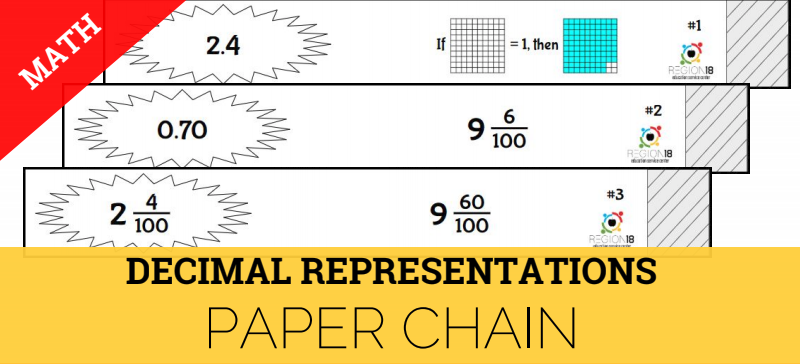Model and relate decimals to fractions that name tenths and hundredths. 4.3D Comparing Fractions Dominoes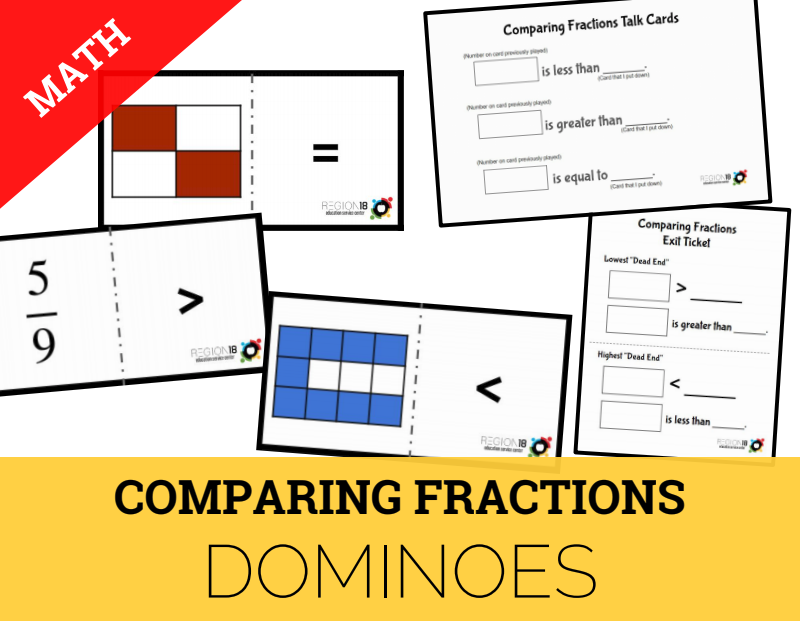Compare two fractions with different numerators and different denominators and represent the comparison using the symbols >, =, or <. 4.3E Adding and Subtracting Fractions Puzzle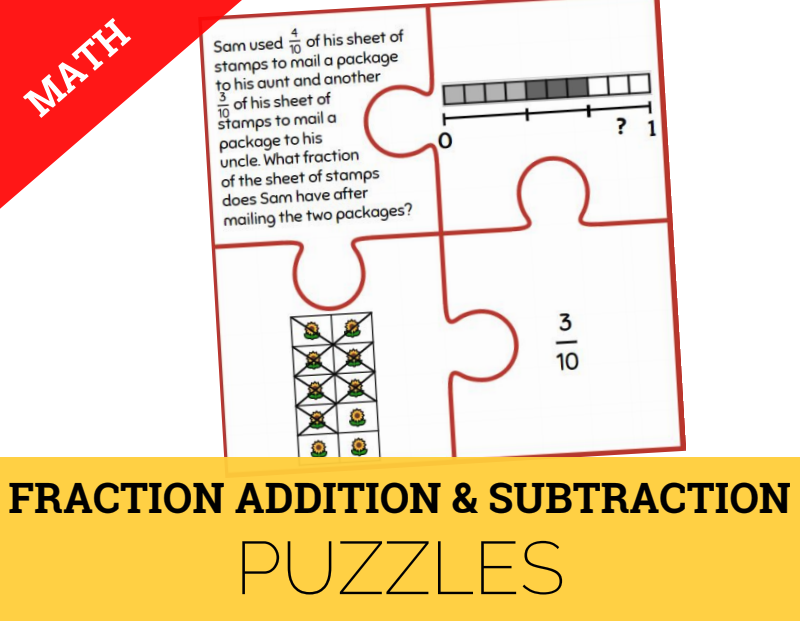Represent and solve addition and subtraction of fractions with equal denominators using objects and pictorial models that build to the number line and properties of operations. 4.4A Addition & Subtraction Word Problems Griddable Practice Task Cards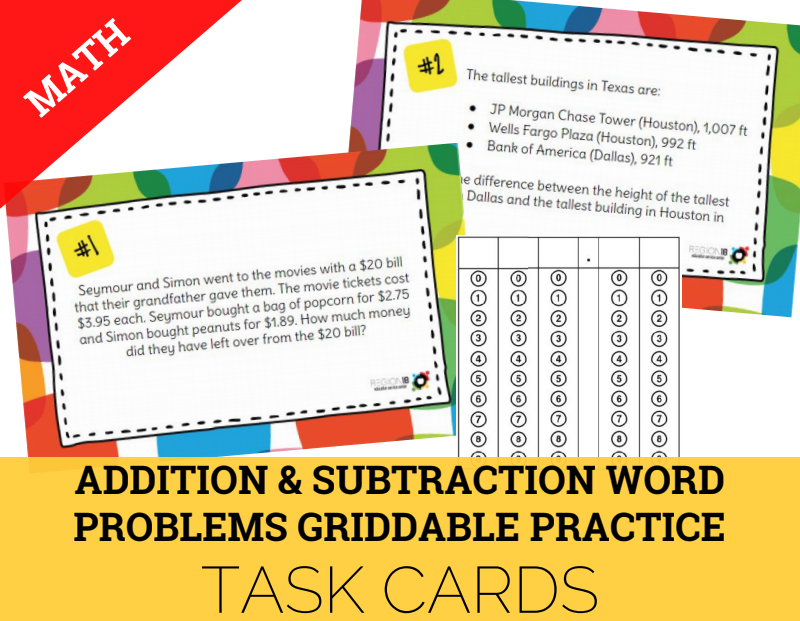Represent and solve division of whole numbers by unit fractions and unit fractions by whole numbers. 4.4H Problem Solving with Multiplication and Division Question Stack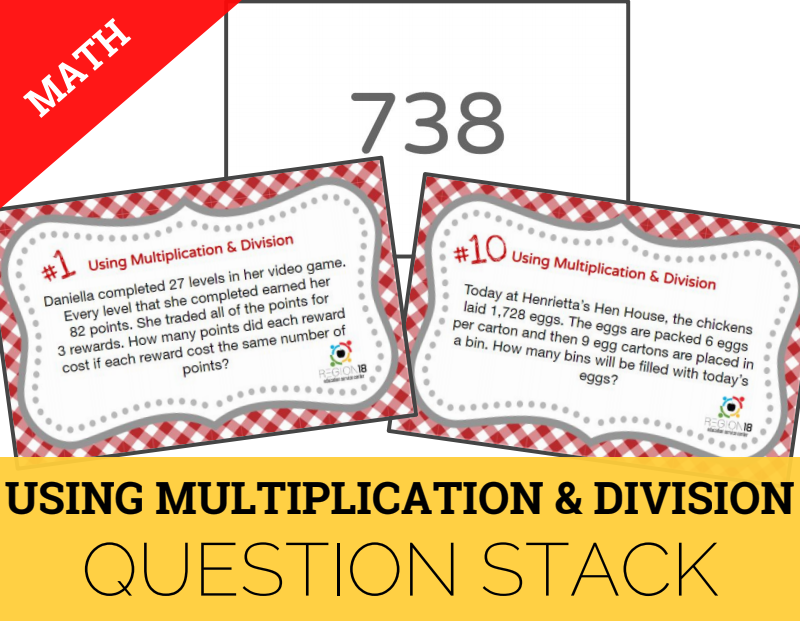Represent and solve multi-step problems involving the four operations with whole numbers using equations with a letter standing for the unknown quantity. 4.5A Multi-Step Problems Using Operations Scavenger Hunt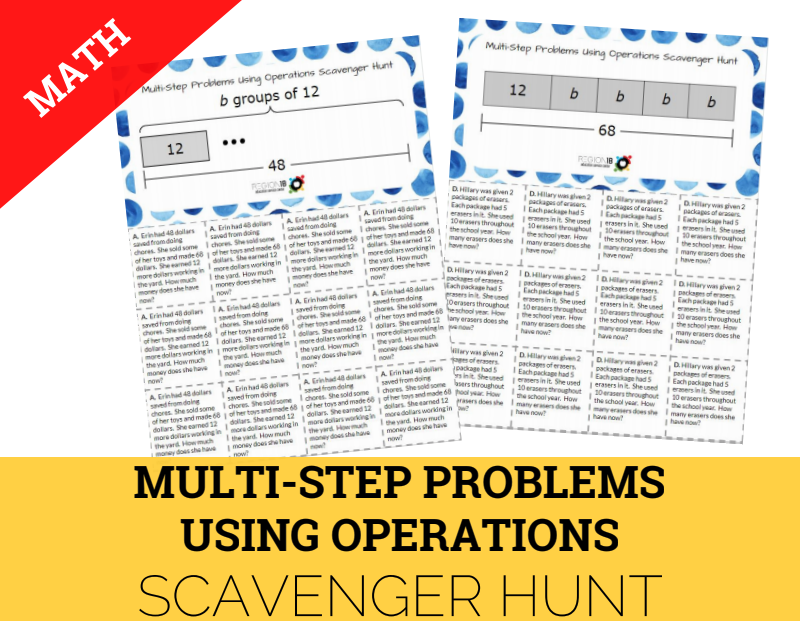Represent multi-step problems involving the four operations with whole numbers using strip diagrams and equations with a letter standing for the unknown quantity. 4.5B Input-Output Tables Match & Snip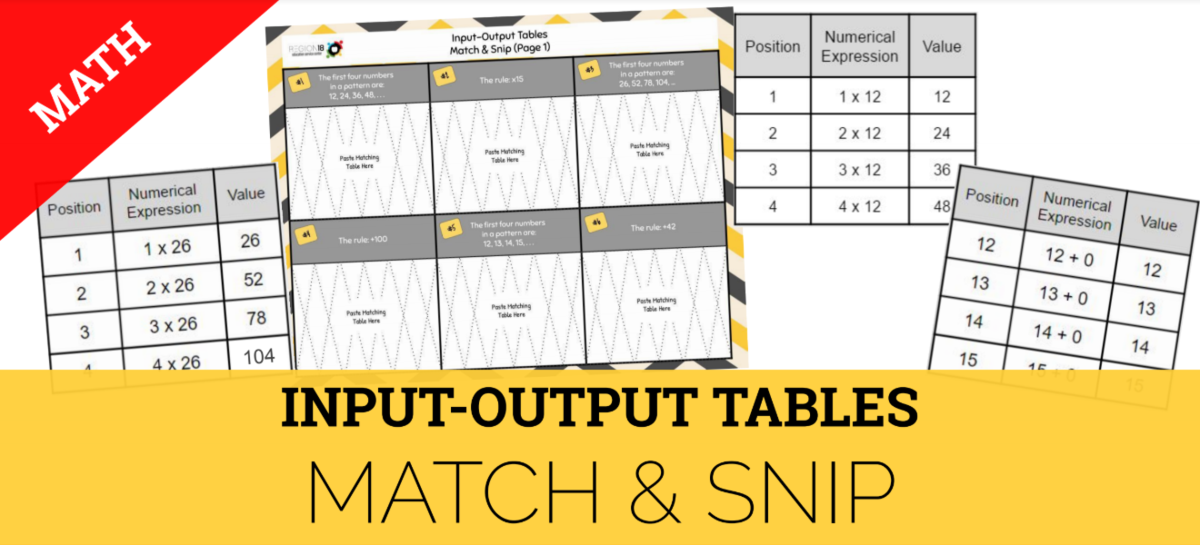Represent problems using an input-output table and numerical expressions to generate a number pattern that follows a given rule representing the relationship of the values in the resulting sequence and their position in the sequence. 4.5D Perimeter & Area Sum It Up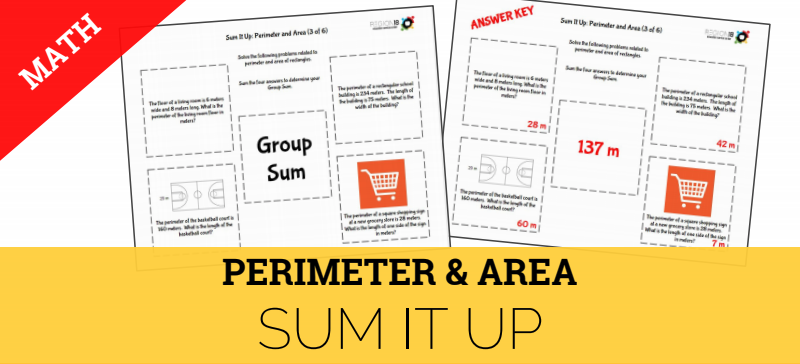Solve problems related to perimeter and area of rectangles where dimensions are whole numbers. 4.6D Attributes of 2D Shapes It's Your Draw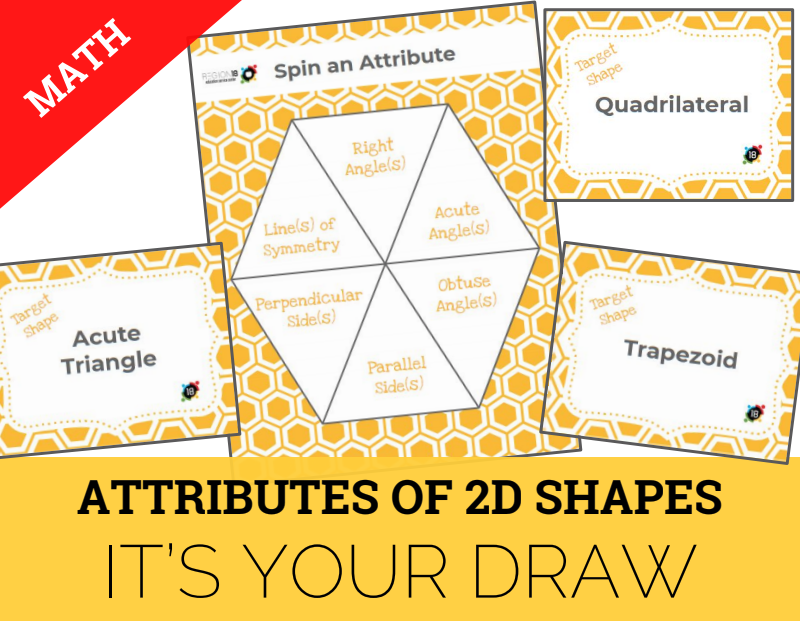Classify two-dimensional figures based on the presence of absence of parallel or perpendicular lines or the presence or absence of angles of a specified size. 4.7C Measuring Angles with Protractors Sum It Up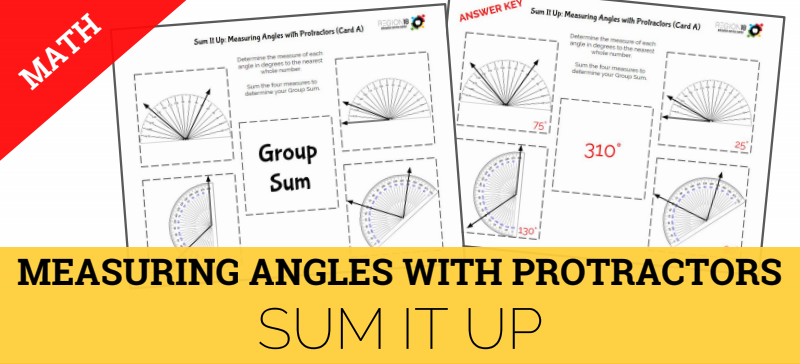Determine the approximate measures of angles in degrees to the nearest whole number using a protractor. 4.8C Problem Solving with Measurement Square Puzzle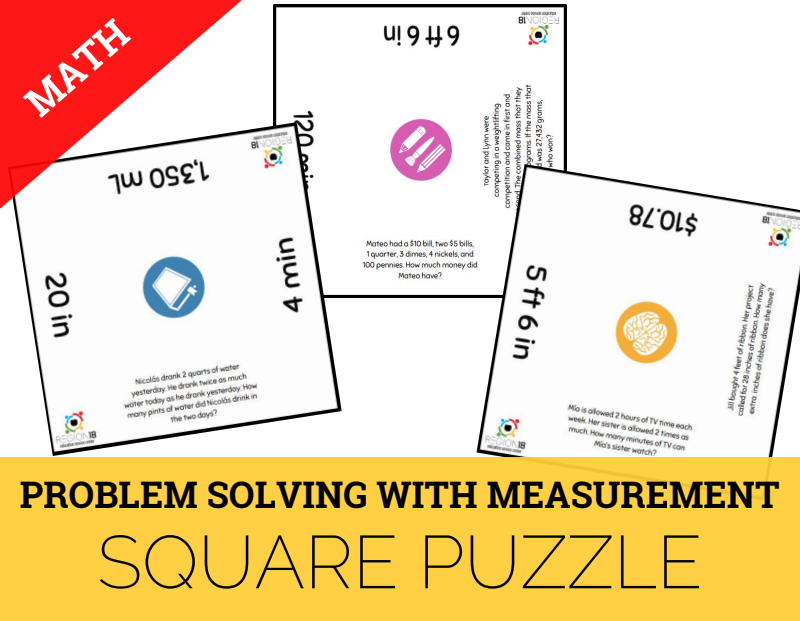Solve problems that deal with measurements of length, intervals of time, liquid volumes, mass, and money using addition, subtraction, multiplication, or division as appropriate. 4.9A Data Representations Card Sort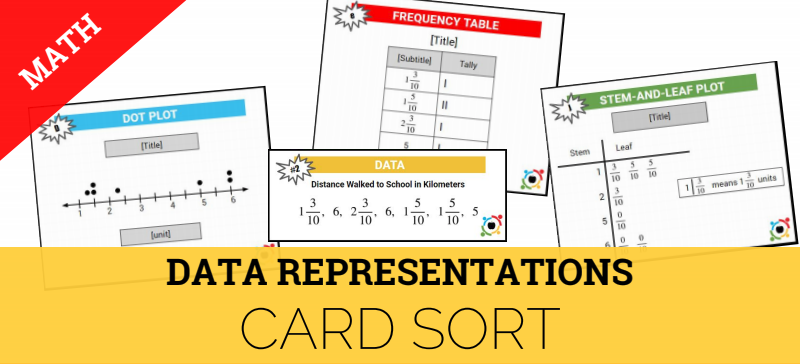Represent data on a frequency table, dot plot, or stem-and-leaf plot marked with whole numbers and fractions.